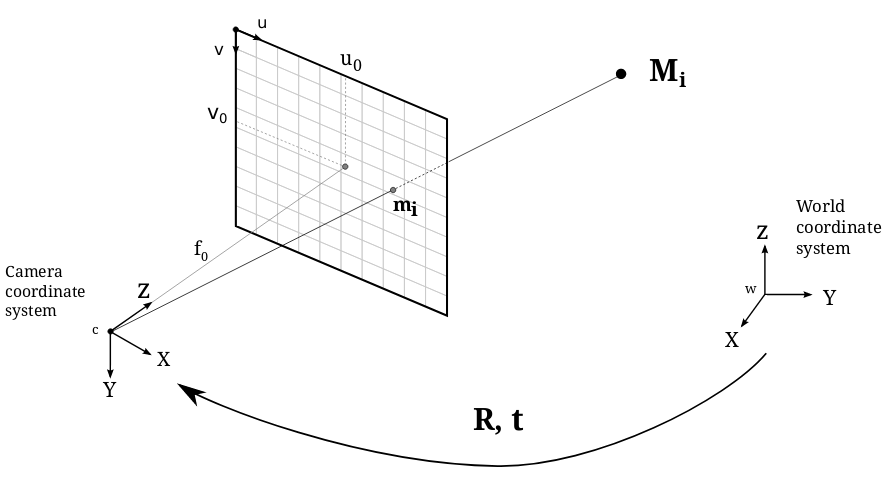# Pinhole Camera¶

In this module we have all the functions and data structures needed to describe the projection of a 3D scene space onto a 2D image plane.

In computer vision, we can map between the 3D world and a 2D image using projective geometry. The module implements the simplest camera model, the Pinhole Camera, which is the most basic model for general projective cameras from the finite cameras group.

The Pinhole Camera model is shown in the following figure:Using this model, a scene view can be formed by projecting 3D points into the image plane using a perspective transformation.

$s \; m' = K [R|t] M'$

or

$\begin{split}s \begin{bmatrix} u \\ v \\ 1\end{bmatrix} = \begin{bmatrix} f_x & 0 & u_0 \\ 0 & f_y & v_0 \\ 0 & 0 & 1 \end{bmatrix} \begin{bmatrix} r_{11} & r_{12} & r_{13} & t_1 \\ r_{21} & r_{22} & r_{23} & t_2 \\ r_{31} & r_{32} & r_{33} & t_3 \end{bmatrix} \begin{bmatrix} X \\ Y \\ Z \\ 1 \end{bmatrix}\end{split}$
where:
• $$M'$$ is a 3D point in space with coordinates $$[X,Y,Z]^T$$ expressed in a Euclidean coordinate system.
• $$m'$$ is the projection of the 3D point $$M'$$ onto the image plane with coordinates $$[u,v]^T$$ expressed in pixel units.
• $$K$$ is the camera calibration matrix, also refered as the instrinsics parameters matrix.
• $$C$$ is the principal point offset with coordinates $$[u_0, v_0]^T$$ at the origin in the image plane.
• $$fx, fy$$ are the focal lengths expressed in pixel units.

The camera rotation and translation are expressed in terms of Euclidean coordinate frame, also known as the world coordinates system. This terms are usually expressed by the joint rotation-translation matrix $$[R|t]$$, or also called as the extrinsics parameters matrix. It is used to describe the camera pose around a static scene and translates the coordinates of a 3D point $$(X,Y,Z)$$ to a coordinate sytstem respect to the camera.